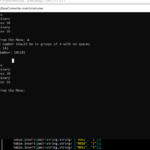## Base Converter In C++ With Source Code

The Base converter is a simple project developed using c++ programming language. The project contains a base conversion system. The user can enter any number and convert them to any number system (like decimal to binary, binary to Hexadecimal, etc.).

Type : Project

File Size : 490.4 KB# Similar Projects and Reports

#### Base Converter In C++ With Source Code

The Base converter is a simple project developed using c++ programming language. The project contains a base conversion system. The user can enter any number and convert them to any number system (like decimal to binary, binary to Hexadecimal, etc.).

#### BASE CONVERTER APP IN PYTHON WITH SOURCE CODE

The Base converter App In Python is a simple project developed using Python. The project contains a base conversion system and calculator. The user can enter any number and convert them to any number systems(like decimal to binary, binary to octal, etc.).

#### Volume Converter In C# With Source Code

This Volume Converter in C# is a simple GUI-based calculation project that is very easy to understand and use. This application converts volume from one unit to another (i.e. centiliter to liter). Also, this project is capable of handling most of the exce...

#### Numerical Base Converter In Python With Source Code

The Numerical Base converter In Python is a simple project developed using Python. The project contains a base conversion system. The user can enter any number and convert them to any number systems(like decimal to binary, binary to octal, etc.).

#### Binary converter In Python with Source code

The Binary converter In Python is a simple project developed using Python. The project contains a base conversion system. The user can enter any number and convert them to other number systems (decimal to binary, binary to decimal).

#### Weight Converter In Python With Source Code

The Weight converter In Python is a simple project developed using Python. This project is a GUI application that converts your weight into different units. Here, you have to enter the weight in kg and the result will be in other different units. And, the...